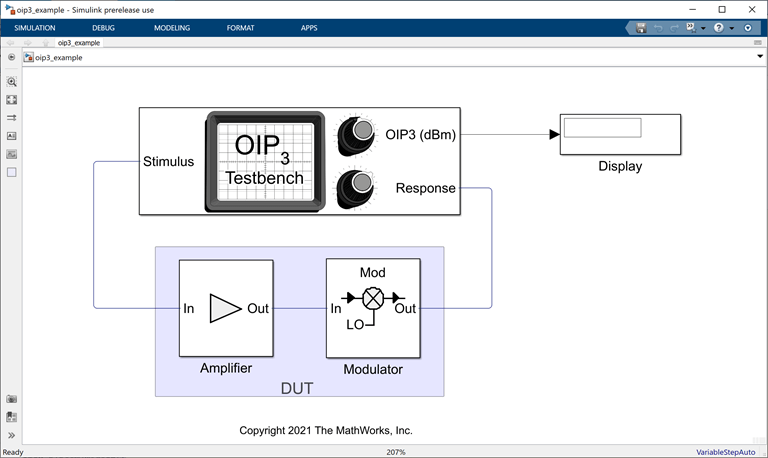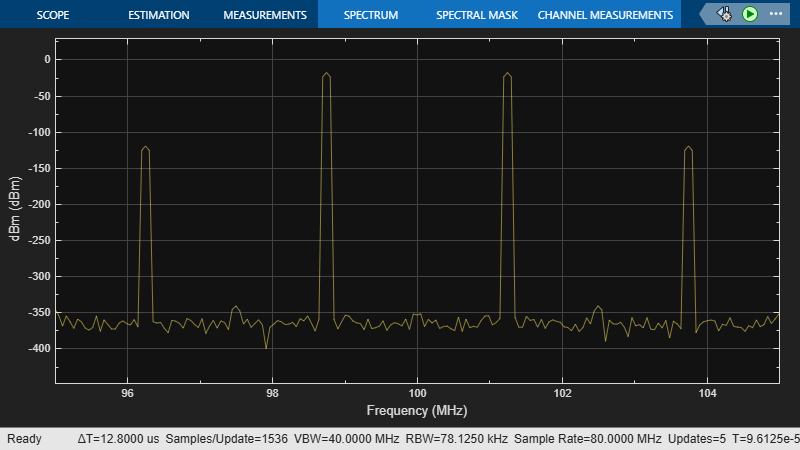# Measure OIP3 of Device Under Test

Use the OIP3 Testbench block to measure the output third order intercept (OIP3) of a device under test (DUT).

Connect the blocks as shown in the model.Set the parameters for DUT and the testbench.

Amplifier block:

• Available power gain`10` dB

• Intercept points convention`Output`

• IP3`32` dBm

Mixer block:

• Available power gain`5` dB

• Local oscillator frequency`2.0` GHz

• Add Image Reject filter`on`

• Intercept points convention`Output`

• IP3`35` dBm

• Filter type`Highpass`

• Implementation`Constant per carrier`

• Passband edge frequency`2.05` GHz

OIP3 Testbench block:

• Input frequency (Hz)`2.1e9`

• Output frequency (Hz)`0.1e9`

• Simulate noise (both stimulus and DUT internal)`off`

Run the model. You will see that the Display block shows an OIP3 value of `32.87` dBm. This value can be verified analytically using the IIP3 equation provided in .

OIP3 of the DUT = IIP3 + Gain = – 10*log10(1/10^((22)/10) + 10^(10/10)/10^((30)/10)) + 15 = 32.8756 dBm

where,

IIP3 of the amplifier in linear scale =10^((32-10)/10), as IIP3 = OIP3 – Gain ; IIP3 = 32 dBm – 10 dB = 22 dBm

IIP3 of the mixer in linear scale = 10^((35-5)/10), as IIP3 = OIP3 – Gain ; IIP3 = 35 dBm – 5 dB = 30 dBm

Available power gain of the amplifier = 10^(10/10)Razavi, Behzad. “Basic Concepts “ in RF Microelectronics, 2nd edition, Prentice Hall, 2012.